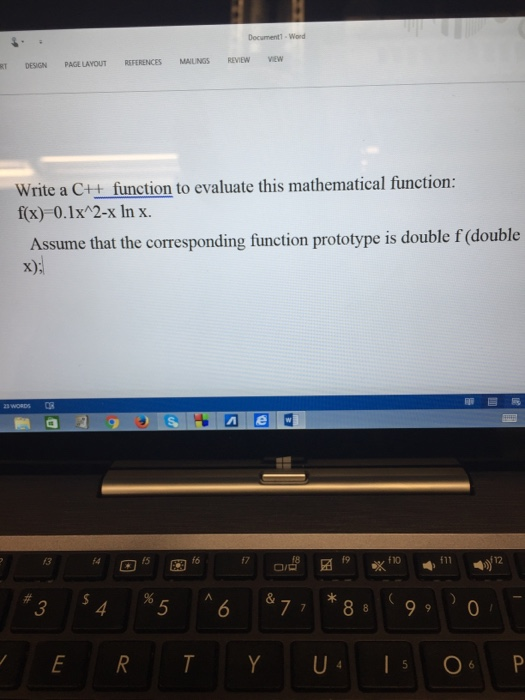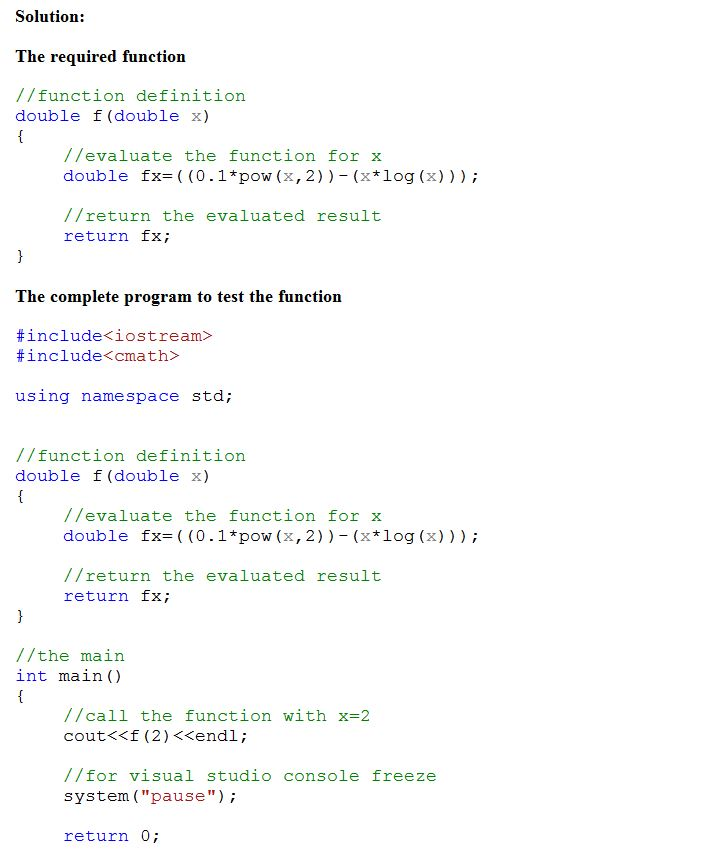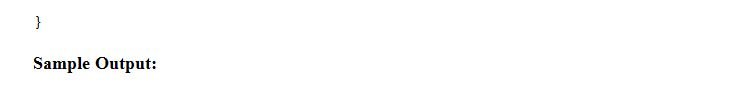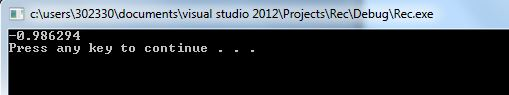# Solved: Write a C++ function to evaluate this mathematical function: f(x) = 0.1x^2-x In x. Assume that the correspondiWrite a C++ function to evaluate this mathematical function: f(x) = 0.1x^2-x In x. Assume that the corresponding function prototype is double f (double x);Don't use plagiarized sources. Get Your Custom Essay on
Solved: Write a C++ function to evaluate this mathematical function: f(x) = 0.1x^2-x In x. Assume that the correspondi
GET AN ESSAY WRITTEN FOR YOU FROM AS LOW AS \$13/PAGE

Raw Paste Function Code:

The required function

//function definition

double f(double x)

{

//evaluate the function for x

double fx=((0.1*pow(x,2))-(x*log(x)));

//return the evaluated result

return fx;

}